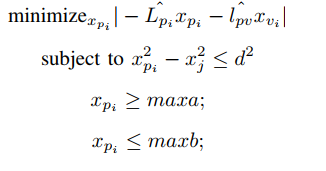# Linear Programming Issue

Hi all,
I am a newbie to CVX tool and trying to implement a problem (Snapshot Attached). I have tried many ways but unable to find the solution. I want to solve it as a Linear Program.

``````lpi_hat =[5 0;0 5];

lpv_hat=[-1 0 -1 0 -1 0 -1 0 -1 0;0 -1 0 -1 0 -1 0 -1 0 -1];
x_v= [1;4;3;4;5;2;3;1;1;1];
x_j=[2;5];
d=[4;4];
cvx_begin
variable x_p(2)
minimize (-lpi_hat.*x_p-lpv_hat.*x_v)
subject to
(x_p)-(x_j)<=d
cvx_end
``````

I am continuously facing a problem like

"Error using .* (line 46)
Matrix dimensions must agree.

Error in CVX_Examplr (line 11)
minimize (-lpi_hat.*x_p-lpv_hat.*x_v)"

I will be quite thankful if someone can help me in this regard.You should not be using `.*`

Perhaps you want
`minimize (norm(-lpi_hat*x_p-lpv_hat*x_v,1))`
or
`minimize (norm(-lpi_hat*x_p-lpv_hat*x_v,inf))`

Using either of these, your program will run without error message. However, You have not correctly implemented the constraint in the image. I believe you want
`x_p.^2 - x_j.^2 < = d^2`
However, that is a convex quadratic constraint, not a linear constraint. It can be solved as is in CVX. . However, it can instead be transformed into two linear (affine) constraints, which when using the one norm or infinity norm in the objective function, will result in a Linear Program.

``````  x_p <= sqrt(x_j.^2 + d^2)
- x_p <= sqrt(x_j.^2 + d^2)
``````

And you forgot to include the constraint
`maxa <= x_p <= maxb`

So the complete program is

``````cvx_begin
variable x_p(2)
minimize (norm(-lpi_hat*x_p-lpv_hat*x_v,1))
x_p <= sqrt(x_j.^2 + d^2)
- x_p <= sqrt(x_j.^2 + d^2)
maxa <= x_p <= maxb
cvx_end
``````

Or perhaps you want
`minimize (norm(-lpi_hat*x_p-lpv_hat*x_v,inf))`
for the objective.

Edit: I changed `d` to `d^2`, so as to match your Snapshot.

1 Like

I am highly thankful for the response. The code perfectly worked for me.